Icosahedron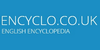A geometrical shape occurring in many virus particles, with 20 triangular faces and 12 corners.

IcosahedronIn geometry, an icosahedron (n or n) is a polyhedron with 20 triangular faces, 30 edges and 12 vertices. A regular icosahedron with identical equilateral faces is often meant because of its geometrical significance as one of the five Platonic solids. It has five triangular faces meeting at each vertex. It can be represented by its vertex figure as...
Found on http://en.wikipedia.org/wiki/Icosahedron

icosahedron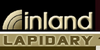Twenty sided polyhedron; all sides are equidimensional. Minerals shaped as icosahedrons belong to the isometric system.
Found on http://www.encyclo.co.uk/local/22291

Icosahedron• (n.) A solid bounded by twenty sides or faces.
Found on http://thinkexist.com/dictionary/meaning/icosahedron/

icosahedron(from the article `cluster`) ...Waals forces or by other simple forces that depend only on the distance between each pair of atoms have unusual stability when the cluster has ... ...and is rapidly decomposed both by water and even by the grease used to lubricate the vacuum equipment employed in its preparation. Closed cages ... ...di...
Found on http://www.britannica.com/eb/a-z/i/5

IcosahedronI`co·sa·he'dron noun [ Greek ...; ... twenty + ... seat, base, from ... to sit.] (Geom.) A solid bounded by twenty sides or faces. Regular icosahedron , one of the five regular polyhedrons, bounded by twenty equilateral triangules. Five triangles meet to form each solid angle of the ...
Found on http://www.encyclo.co.uk/webster/I/3

IcosahedronA 20 sided solid.
Found on http://www.diracdelta.co.uk/science/source/i/c/icosahedron/source.html

icosahedronA geometrical shape occurring in many virus particles, with 20 triangular faces and 12 corners. ... (09 Oct 1997) ...
Found on http://www.encyclo.co.uk/local/20973

icosahedronA polyhedron with 20 faces. A regular icosahedron has faces that are all equilateral triangles, and is one of the five Platonic solids. The length from vertex to opposing vertex of a regular icosahedron is 51/4 f1/2 d where f (phi) is the golden ...
Found on http://www.daviddarling.info/encyclopedia/I/icosahedron.html

Icosahedron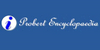An icosahedron is a solid with twenty sides or faces. A regular icosahedron is one of the five regular polyhedrons, bounded by twenty equilateral triangles. Five triangles meet to form each solid angle of the polyhedron.
Found on http://www.probertencyclopaedia.com/browse/GI.HTM

icosahedron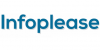icosahedron (īkō"suhē'drun) : see polyhedron.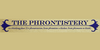solid with twenty plane faces[n] - any polyhedron having twenty plane faces